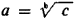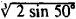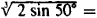# Logarithm, Taking The

The following article is from The Great Soviet Encyclopedia (1979). It might be outdated or ideologically biased.

## Logarithm, Taking The

the operation of finding the logarithm of a numerical, algebraic, or other expression. Taking the logarithm is one of two operations that are the inverse of raising to a power. If ab = c, thenand b = logac. Taking the logarithm is used in practical computations to reduce the operations of multiplication, division, raising to a power, and extraction of a root to the operations of addition, subtraction, multiplication, and division. For example, in order to approximatewe may use the relationship log(1/3) log 2 + (1/3) log sin 50° and then tables of logarithms.